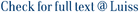Given a directed graph, two vertices v and w are 2-vertex-connected if there are two internally vertex-disjoint paths from v to w and two internally vertex-disjoint paths from w to v. In this paper, we show how to compute this relation in O(m + n) time, where n is the number of vertices and m is the number of edges of the graph. As a side result, we show how to build in linear time an O(n)-space data structure, which can answer in constant time queries on whether any two vertices are 2-vertex-connected. Additionally, when two query vertices v and w are not 2-vertex-connected, our data structure can produce in constant time a “witness” of this property, by exhibiting a vertex or an edge that is contained in all paths from v to w or in all paths from w to v.

2-vertex connectivity in directed graphs / Georgiadis, L; Italiano, Giuseppe Francesco; Laura, L; Parotsidis, N. - In: INFORMATION AND COMPUTATION. - ISSN 0890-5401. - 261:(2018), pp. 248-264. [10.1016/j.ic.2018.02.007]

### 2-vertex connectivity in directed graphs

#### Abstract

Given a directed graph, two vertices v and w are 2-vertex-connected if there are two internally vertex-disjoint paths from v to w and two internally vertex-disjoint paths from w to v. In this paper, we show how to compute this relation in O(m + n) time, where n is the number of vertices and m is the number of edges of the graph. As a side result, we show how to build in linear time an O(n)-space data structure, which can answer in constant time queries on whether any two vertices are 2-vertex-connected. Additionally, when two query vertices v and w are not 2-vertex-connected, our data structure can produce in constant time a “witness” of this property, by exhibiting a vertex or an edge that is contained in all paths from v to w or in all paths from w to v.
##### Scheda breve Scheda completa Scheda completa (DC)2018
2-vertex connectivity in directed graphs / Georgiadis, L; Italiano, Giuseppe Francesco; Laura, L; Parotsidis, N. - In: INFORMATION AND COMPUTATION. - ISSN 0890-5401. - 261:(2018), pp. 248-264. [10.1016/j.ic.2018.02.007]
File in questo prodotto:
File

Solo gestori archivio

Tipologia: Versione dell'editore
Licenza: DRM (Digital rights management) non definiti
Dimensione 973.46 kB
Utilizza questo identificativo per citare o creare un link a questo documento: `https://hdl.handle.net/11385/182810`
•10
•5